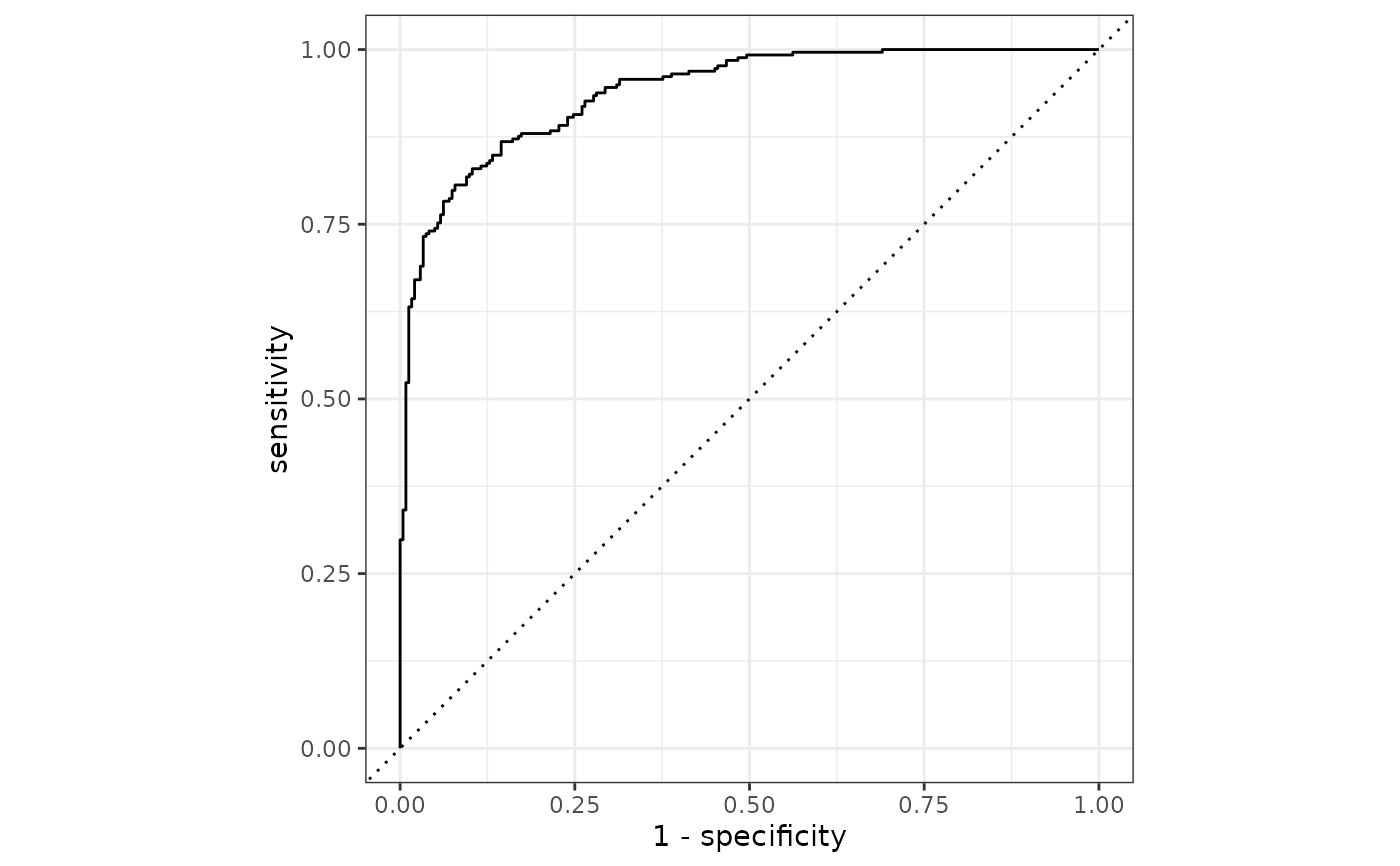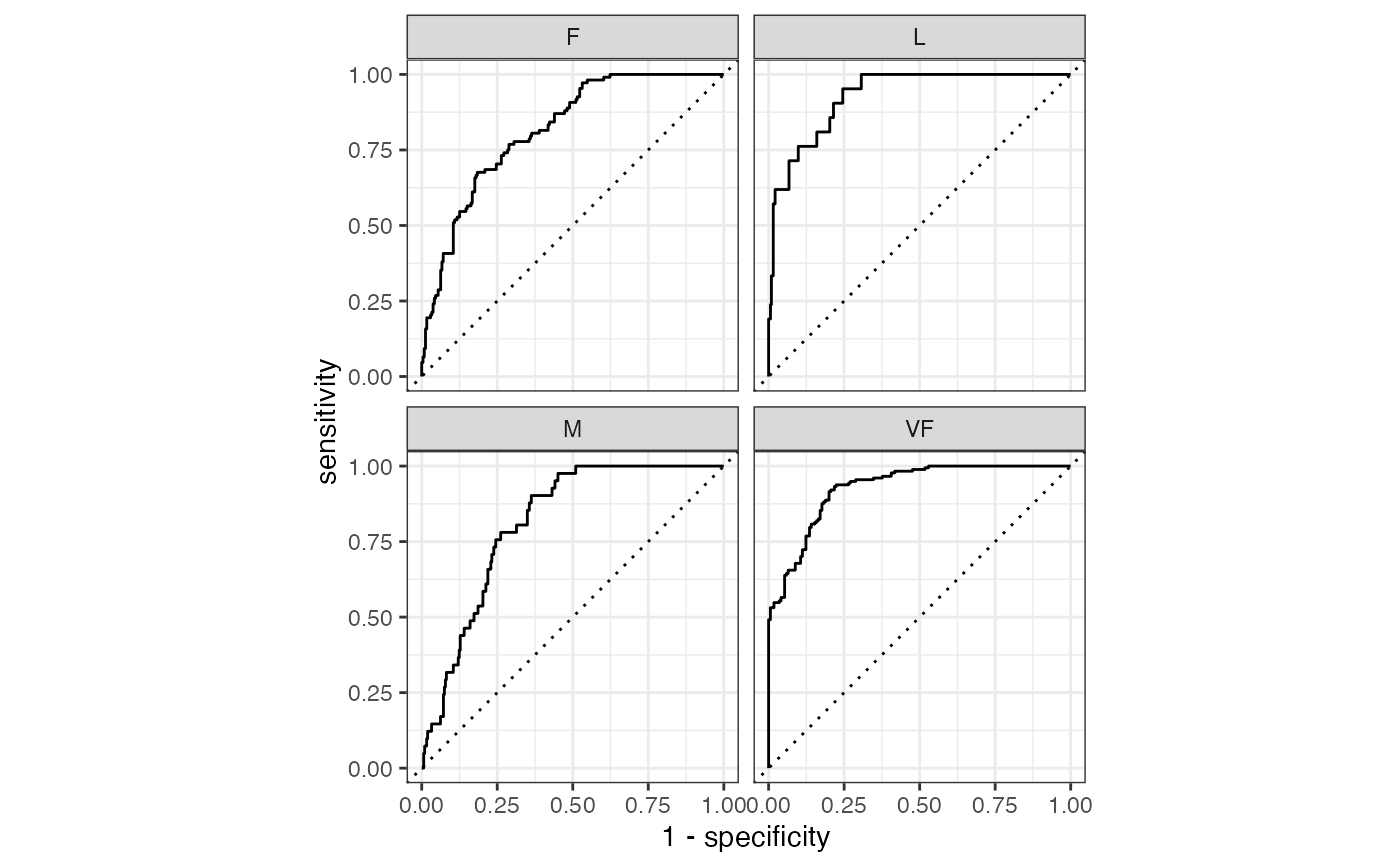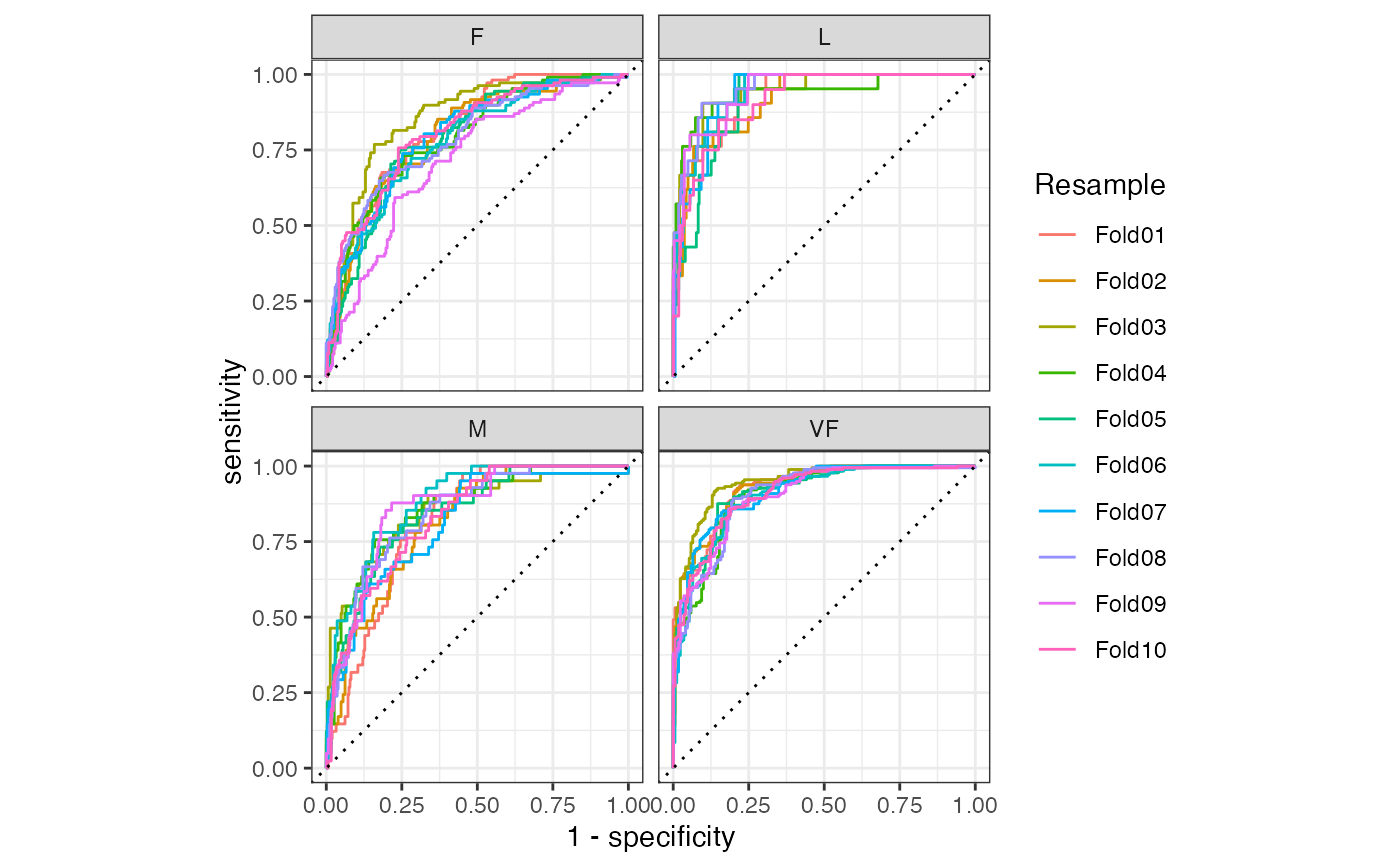roc_curve() constructs the full ROC curve and returns a tibble. See roc_auc() for the area under the ROC curve.

roc_curve(data, ...)

# S3 method for data.frame
roc_curve(data, truth, ..., options = list(), na_rm = TRUE)

autoplot.roc_df(object, ...)

## Arguments

data A data.frame containing the truth and estimate columns. A set of unquoted column names or one or more dplyr selector functions to choose which variables contain the class probabilities. If truth is binary, only 1 column should be selected. Otherwise, there should be as many columns as factor levels of truth. The column identifier for the true class results (that is a factor). This should be an unquoted column name although this argument is passed by expression and supports quasiquotation (you can unquote column names). For _vec() functions, a factor vector. A list of named options to pass to pROC::roc() such as direction or smooth. These options should not include response, predictor, levels, or quiet. A logical value indicating whether NA values should be stripped before the computation proceeds. The roc_df data frame returned from roc_curve().

## Value

A tibble with class roc_df or roc_grouped_df having columns specificity and sensitivity.

If an ordinary (i.e. non-smoothed) curve is used, there is also a column for .threshold.

## Details

roc_curve() computes the sensitivity at every unique value of the probability column (in addition to infinity and minus infinity). If a smooth ROC curve was produced, the unique observed values of the specificity are used to create the curve points. In either case, this may not be efficient for large data sets.

There is a ggplot2::autoplot() method for quickly visualizing the curve. This works for binary and multiclass output, and also works with grouped data (i.e. from resamples). See the examples.

## Multiclass

If a multiclass truth column is provided, a one-vs-all approach will be taken to calculate multiple curves, one per level. In this case, there will be an additional column, .level, identifying the "one" column in the one-vs-all calculation.

## Relevant Level

There is no common convention on which factor level should automatically be considered the "event" or "positive" result. In yardstick, the default is to use the first level. To change this, a global option called yardstick.event_first is set to TRUE when the package is loaded. This can be changed to FALSE if the last level of the factor is considered the level of interest by running: options(yardstick.event_first = FALSE). For multiclass extensions involving one-vs-all comparisons (such as macro averaging), this option is ignored and the "one" level is always the relevant result.

Compute the area under the ROC curve with roc_auc().

Other curve metrics: gain_curve(), lift_curve(), pr_curve()

## Examples

# ---------------------------------------------------------------------------
# Two class example

# truth is a 2 level factor. The first level is "Class1", which is the
# "event of interest" by default in yardstick. See the Relevant Level
# section above.
data(two_class_example)

# Binary metrics using class probabilities take a factor truth column,
# and a single class probability column containing the probabilities of
# the event of interest. Here, since "Class1" is the first level of
# "truth", it is the event of interest and we pass in probabilities for it.
roc_curve(two_class_example, truth, Class1)#> # A tibble: 502 x 3
#>    .threshold specificity sensitivity
#>         <dbl>       <dbl>       <dbl>
#>  1 -Inf           0                 1
#>  2    1.79e-7     0                 1
#>  3    4.50e-6     0.00413           1
#>  4    5.81e-6     0.00826           1
#>  5    5.92e-6     0.0124            1
#>  6    1.22e-5     0.0165            1
#>  7    1.40e-5     0.0207            1
#>  8    1.43e-5     0.0248            1
#>  9    2.38e-5     0.0289            1
#> 10    3.30e-5     0.0331            1
#> # … with 492 more rows
# ---------------------------------------------------------------------------
# autoplot()

# Visualize the curve using ggplot2 manually
library(ggplot2)
library(dplyr)
roc_curve(two_class_example, truth, Class1) %>%
ggplot(aes(x = 1 - specificity, y = sensitivity)) +
geom_path() +
geom_abline(lty = 3) +
coord_equal() +
theme_bw()# Or use autoplot
autoplot(roc_curve(two_class_example, truth, Class1))# \dontrun{

# Multiclass one-vs-all approach
# One curve per level
hpc_cv %>%
filter(Resample == "Fold01") %>%
roc_curve(obs, VF:L) %>%
autoplot()# Same as above, but will all of the resamples
hpc_cv %>%
group_by(Resample) %>%
roc_curve(obs, VF:L) %>%
autoplot()# }